polarplots2.mws

> with(plots):

Polar Plots

1. Plot the graph of r = sin(2theta) for theta in [0, 2Pi]

> polarplot(sin(2*theta), theta=0..4*Pi, title="r = sin(2 Theta)");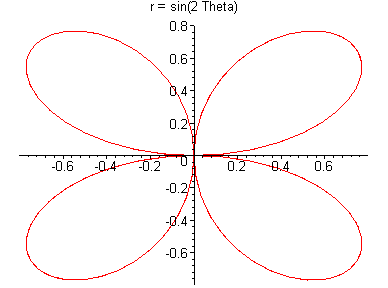> 2. Plot the graph of r = theta for theta in [0, 2Pi]

> polarplot(theta, theta=0..2*Pi, title = "r = Theta, Spiral of Archimedes");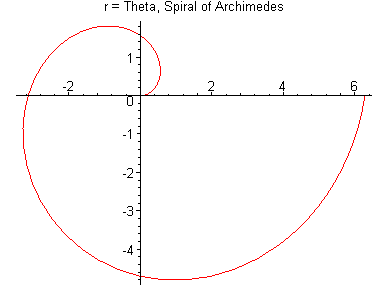3. Plot the graph of r = exp(-2theta) for theta in [0, 2Pi]

> polarplot(exp(-2*Theta), Theta=0.. 5*Pi, title="r = exp(-2 Theta), asymptotic to zero");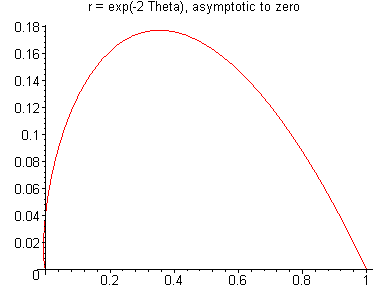4. Plot the graph of r = 1-2cos(sin(theta)) for theta in [0, 2Pi]

> polarplot(1-2*cos(sin(theta)), theta=0..2*Pi, title="Goofy eyes: r = 1 - 2cos(sin(theta))");

5. Plot the graph of r = 1-cos(sin(theta)) for theta in [0, 2Pi]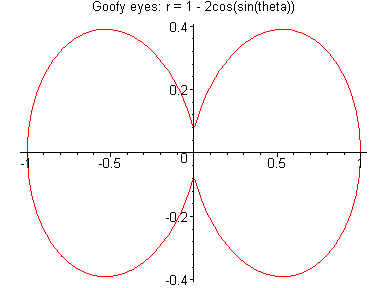> polarplot(1-cos(sin(theta)), theta=0..2*Pi, title="Figure-eight: r = 1 - cos(sin(theta))");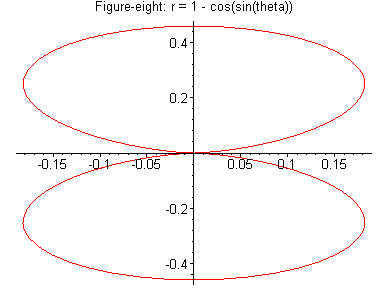6. Plot the graph of r = 1+cos(theta) for theta in [0, 2Pi]. This is a cardioid...

> polarplot(1+cos(x), x=0..24*Pi, title="r = 1+cos(theta)");

7. Plot the graph of r = 1+2cos(theta) for theta in [0, 2Pi]. This is a cardioid with an inner loop...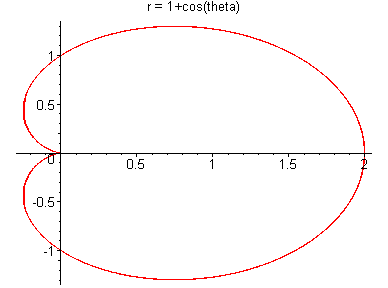> polarplot(1+2*cos(x), x=0..2*Pi, title="r = 1+2cos(theta)");

8. Plot the graph of r = sin(3theta) for theta in [0, 2Pi]. This has three petals ...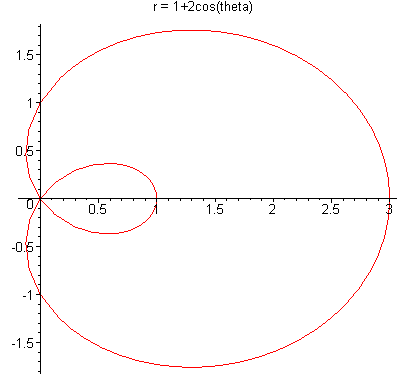> polarplot(sin(3*theta), theta=0..2*Pi, title="r = sin(3Theta)");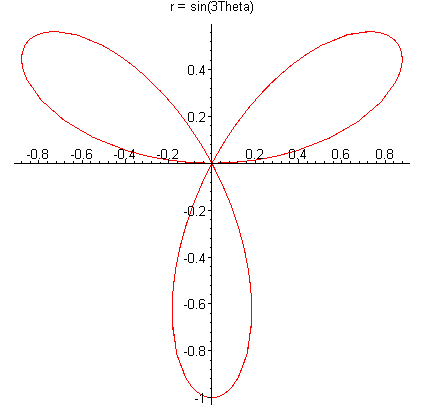9. Plot the graph of r = cos(3theta) for theta in [0, 2Pi]. This has three petals, but rotated ...compare with the preceding polarplot(cos(3*theta), theta=0..2*Pi, title="r = cos(3Theta)");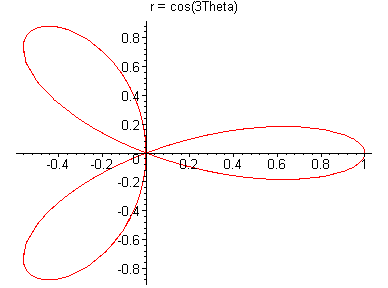> 10. Plot the graph of r = 1 + 2cos(theta) for theta in [0, 2Pi]. This has three petals, but rotated ...compare with the preceding

> polarplot(1+2*cos(x), x=0..2*Pi, title="r = 1+2cos(theta)");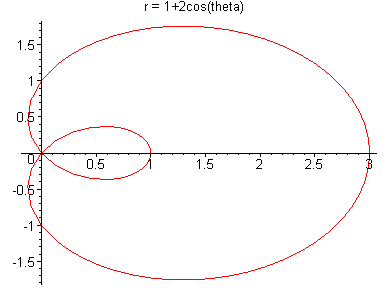> polarplot(sin(sqrt(2)*theta)+cos(theta), theta=0..3*Pi, title="r = sin (sqrt(2) theta) + cos(theta). A quasi-periodic plot");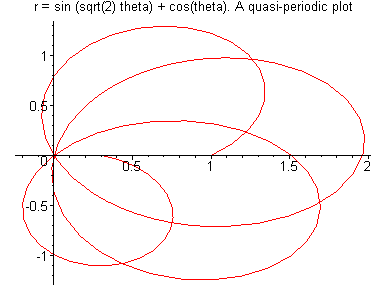>

>